# ML Aggarwal Class 10 Solutions for ICSE Maths Chapter 16 Constructions Chapter Test

## ML Aggarwal Class 10 Solutions for ICSE Maths Chapter 16 Constructions Chapter Test

ML Aggarwal Class 10 Solutions for ICSE Maths Chapter 16 Constructions Chapter Test

Question 1.
Draw a circle of radius 3 cm. Mark its centre as C and mark a point P such that CP = 7 cm. Using a ruler and compasses only, Construct two tangents from P to the circle.
Solution: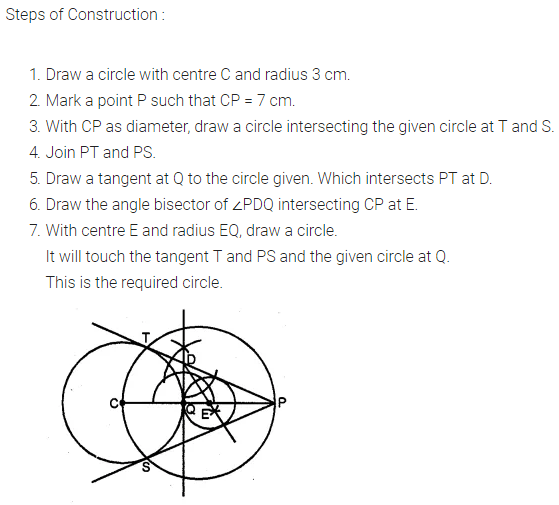Question 2.
Draw a line AQ = 7 cm. Mark a point P on AQ such that AP = 4 cm. Using a ruler and compasses only, construct:
(i) a circle with AP as diameter.
(ii) two tangents to the above circle from the point Q.
Solution: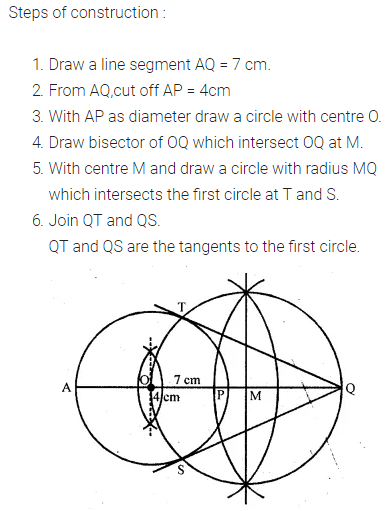Question 3.
Using ruler and compasses only, construct a triangle ABC having given c = 6 cm, b = 1 cm and ∠A = 30°. Measure side a. Draw carefully the circumcircle of the triangle.
Solution: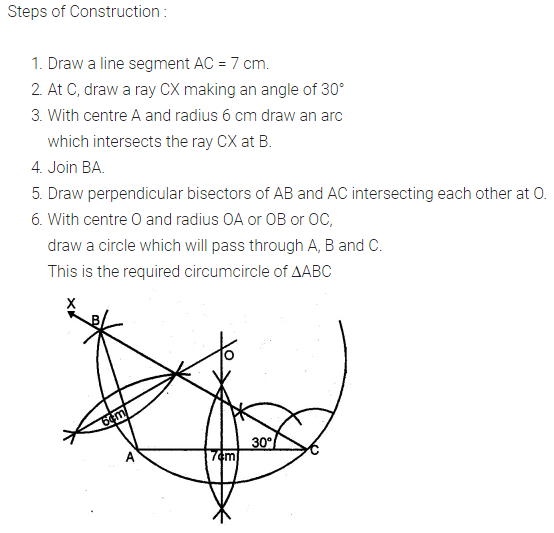Question 4.
Using ruler and compasses only, construct an equilateral triangle of height 4 cm and draw its circumcircle.
Solution: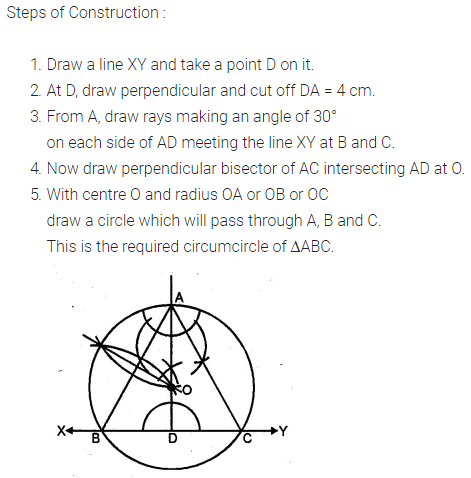Question 5.
Using a ruler and compasses only:
(i) Construct a triangle ABC with the following data: BC = 7 cm, AB = 5 cm and ∠ABC = 45°.
(ii) Draw the inscribed circle to ∆ABC drawn in part (i).
Solution: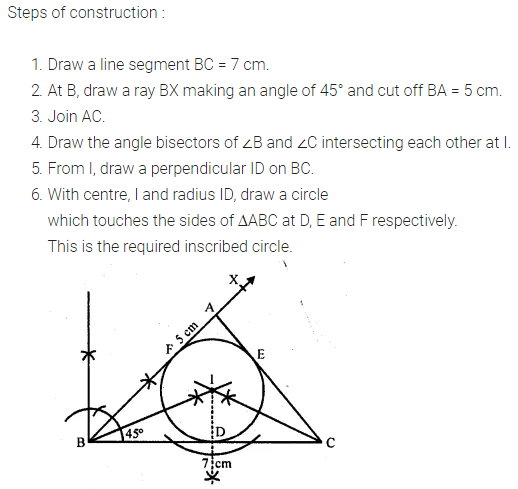Question 6.
Draw a triangle ABC, given that BC = 4 cm, ∠C = 75° and that radius of the circumcircle of ∆ABC is 3 cm.
Solution:Question 7.
Draw a regular hexagon of side 3.5 cm construct its circumcircle and measure its radius.
Solution: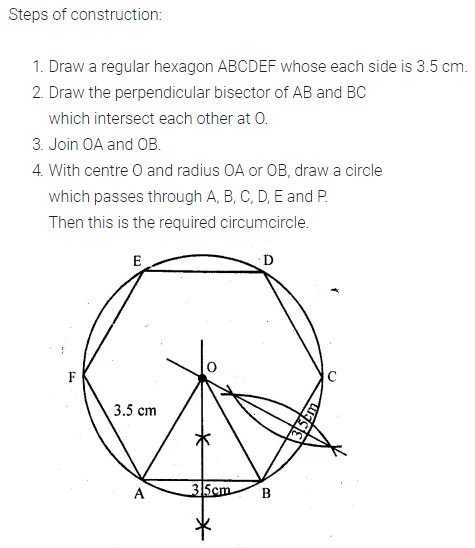Question 8.
Construct a triangle ABC with the following data: AB = 5 cm, BC = 6 cm and ∠ABC = 90°.
(i) Find a point P which is equidistant from B and C and is 5 cm from A. How many such points are there?
(ii) Construct a circle touching the sides AB and BC, and whose centre is equidistant from B and C.
Solution:ML Aggarwal Class 10 Solutions for ICSE Maths